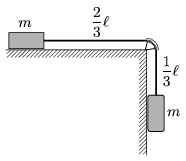Mathematical and Physical Journal
for High Schools
Issued by the MATFUND Foundation
 Already signed up? New to KöMaL?

#Problem P. 3985. (May 2007)

P. 3985. Two objects of mass m are connected with a rope of lengthas shown in the figure. The rope can slide in a tube which forms a quarter of a circle, and which is fixed to the edge of the table. Initiallyof the rope hangs vertically. Friction is negligible.a) If the system is released, how long does it take for the object on the right handside to descend a distance of, and at that time what are the speeds of the objects?

b) What would be the speeds of the object after covering the same distance if the mass of the rope was m as well?

(4 pont)

Deadline expired on June 11, 2007.

### Statistics:

 108 students sent a solution. 4 points: 52 students. 3 points: 12 students. 2 points: 22 students. 1 point: 7 students. 0 point: 5 students. Unfair, not evaluated: 10 solutionss.

Problems in Physics of KöMaL, May 2007